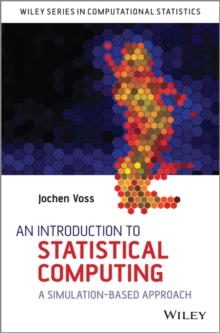Supporting your high street Find out how »
• My AccountAn Introduction to Statistical Computing : A Simulation-Based Approach Hardback

Part of the Wiley Series in Computational Statistics series

Description

A comprehensive introduction to sampling-based methods in statistical computing The use of computers in mathematics and statistics has opened up a wide range of techniques for studying otherwise intractable problems.

Sampling-based simulation techniques are now an invaluable tool for exploring statistical models.

This book gives a comprehensive introduction to the exciting area of sampling-based methods.

An Introduction to Statistical Computing introduces the classical topics of random number generation and Monte Carlo methods.

It also includes some advanced methods such as the reversible jump Markov chain Monte Carlo algorithm and modern methods such as approximate Bayesian computation and multilevel Monte Carlo techniques An Introduction to Statistical Computing: * Fully covers the traditional topics of statistical computing. * Discusses both practical aspects and the theoretical background. * Includes a chapter about continuous-time models. * Illustrates all methods using examples and exercises. * Provides answers to the exercises (using the statistical computing environment R); the corresponding source code is available online. * Includes an introduction to programming in R. This book is mostly self-contained; the only prerequisites are basic knowledge of probability up to the law of large numbers.

Careful presentation and examples make this book accessible to a wide range of students and suitable for self-study or as the basis of a taught course

Information

• Format: Hardback
• Pages: 396 pages
• Publisher: John Wiley & Sons Inc
• Publication Date:
• Category: Probability & statistics
• ISBN: 9781118357729

£59.25

£50.55

on all orders

Pick up orders

from local bookshops

£72.00

£61.20

£72.00

£61.20

£105.00

£93.55

£111.00

£102.55